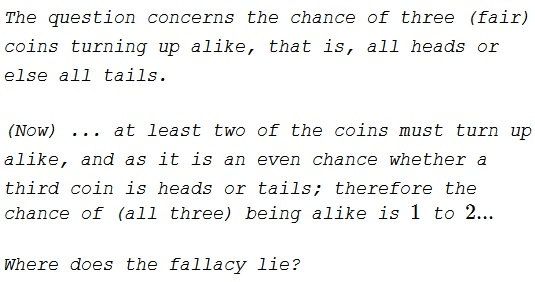### Problem### Solution

First, this is a fallacy. A experiment with three fair coins have eight equiprobable outcomes, so each comes up with the probability of $\displaystyle \frac{1}{8}.$ Two of them - $HHH$ or $TTT$ - combine into an event of the probability $\displaystyle \frac{1}{8}+\frac{1}{8}=\frac{1}{4},$ not $\displaystyle \frac{1}{2}.$ Thus the question: Where does the fallacy lie?

The argument is valid to a point. There are indeed always two coins that come up the same way: both heads up or both tails up. (This is a trivial application of the Pigeonhole Principle.) It is also true that the remaining coin comes up either way with the probability of $\displaystyle \frac{1}{2}.$ The conclusion is, however, false because there is no certainty of which two coins get involved in the first part of the argument. If the two coins were fixed, say, #1 and #2, then certainly the third one - #3 - could come either way with the probability of $\displaystyle \frac{1}{2}.$ But, in that case, #1 and #2 may come up alike with the probability of $\displaystyle 2\cdot\frac{1}{2}\cdot\frac{1}{2}=\frac{1}{2},$ for all four possible outcomes $HH,$ $HT,$ $TH,$ $TT$ are equiprobable. Thus there is no certainty that #1 and #2 will come up alike.

Among eight equiprobable outcomes of three tosses of a coin, exactly two come up alike with the probability of $\displaystyle \frac{3}{4},$ but there is no knowing which pair it is:

$HHH,\underline{HHT},\underline{HTH},\underline{HTT},\underline{THH},\underline{THT},\underline{TTH},TTT.$

### Acknowledgment

This is a problem from Ruma Falk's Understanding Probability and Statistics (A K Peters/CRC Press, 1993, 2.1.10) The paradox is named after Francis Galton who published it in 1894. The problem is formulated in his own words.